# Approximating ∏ With a Coin!

## Homework Statement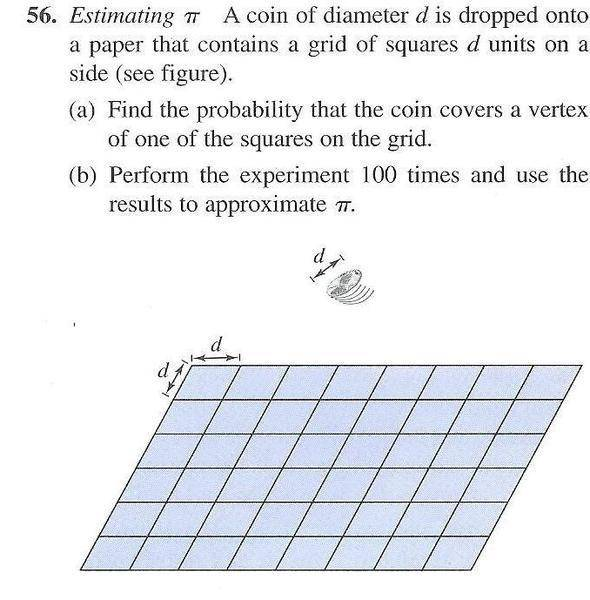## The Attempt at a Solution

I found this interesting problem in my textbook. I have heard of Buffon's needle experiment which estimates pi through probability. This problem came from a pre-calc book out of the probability section. The problem seams similar to Buffon's needle in that d is the diameter of the coin as well as the distance in between each square. However, this is a coin and a grid, not parallel lines and a needle. I'm not sure whether the question is asking for an analytical solution to b or an actual experiment. Can it be determined analytically?

The probability for hitting a vertex would be the same for any d value chosen. I believe it would be much more likely to hit a vertex than to land exactly in between two parallel lines. Does finding the probability involve the area taken up if a coin were placed in each square grid, and the free space left?

#### Attachments

vela
Staff Emeritus
Homework Helper
I found this interesting problem in my textbook. I have heard of Buffon's needle experiment which estimates pi through probability. This problem came from a pre-calc book out of the probability section. The problem seams similar to Buffon's needle in that d is the diameter of the coin as well as the distance in between each square. However, this is a coin and a grid, not parallel lines and a needle. I'm not sure whether the question is asking for an analytical solution to b or an actual experiment. Can it be determined analytically?
It's asking you to do an experiment, but you also need the analytical solution to know how to get the estimate for pi.
The probability for hitting a vertex would be the same for any d value chosen. I believe it would be much more likely to hit a vertex than to land exactly in between two parallel lines. Does finding the probability involve the area taken up if a coin were placed in each square grid, and the free space left?
Think about where the center of the coin can land so that the coin will cover a corner.

Office_Shredder
Staff Emeritus
Gold Member
would be much more likely to hit a vertex than to land exactly in between two parallel lines. Does finding the probability involve the area taken up if a coin were placed in each square grid, and the free space left?

It doesn't need to land exactly in between two parallel lines

As an example I made a picture

http://img138.imageshack.us/img138/7943/circleinsquare.png [Broken]

As for how to go about solving the probability exactly, vela has good advice

Last edited by a moderator:
Think about where the center of the coin can land so that the coin will cover a corner.

Would this be true?: If you were to inscribe a circle of d diameter inside a square with sides length d, the center of a coin with diameter d tracing the circumference the circle would touch a corner at every position on the circle except when the coin is situated at angles 0,90,180,360 on the d diameter circle.

vela
Staff Emeritus
Homework Helper
If I understand what you're saying, the answer is yes. In the four cases you mentioned, the coin would intersect with two corners simultaneously.

DaveC426913
Gold Member
It does not matter if the circle lands on a gridline. What matters is when the circle lands on TWO gridlines simultaneously. (i.e. it will be covering a vertex where two lines intersect).

That is the crux of the experiment.

Think about where the center of the coin can land so that the coin will cover a corner.

When you say a corner do you mean an intersection of only 2 lines or an intersection of 2 or more lines?

Office_Shredder
Staff Emeritus
Gold Member
Where do more than 2 lines intersect on a grid?

O I see. I was considering each side of the square to be a line, but your considering the movement across the grid in the x and y directions as a whole to be lines.

Ok, so I thought I'd make some pictures to illustrate my thinking.

You can have intersection like this...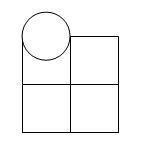You can move that circle anywhere on the x-axis and it will cover 2 intersecting lines. You can also have the same thing but on the y-axis and moving that up and down anywhere will cover 2 intersecting lines with every possible placement along that axis.

Then you can move the circle just a little bit in the x any y directions and it wont cover 2 intersecting lines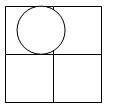Then you can move the circle diagonally and it will intersect.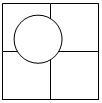Am I making this more complicated than it is? Because is seams like there is a whole lot of possibilities for when it is touching and when it isn't.

#### Attachments

vela
Staff Emeritus
Homework Helper
Concentrate on the middle intersection in your pictures. Where does the center of the circle have to be in relation to it for it to lie within the circle?

well if you drew a circle of d diameter around the center intersection

and had another circle of d diameter trace around the circle with it's center point, it would always touch the center point on the intersection no matter where along the circle it is.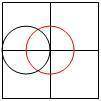#### Attachments

Office_Shredder
Staff Emeritus
Gold Member
well if you drew a circle of d diameter around the center intersection

and had another circle of d diameter trace around the circle with it's center point, it would always touch the center point on the intersection no matter where along the circle it is.That's good. Now, given a square, where can the center be in that square so that the circle hits one of the corners of the square?

That's good. Now, given a square, where can the center be in that square so that the circle hits one of the corners of the square?

It would look like this.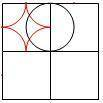The center of the circle would be able to travel along thoes lines and hit an intersection. Infact the only place it wont hit an intersection is in the center area in the square. If the center of the circle is in that center area it wont touch an intersection. Would you need to find the area of the center area verses the area of the outside parts and set up a ratio.

Well you can calculate the inside area to be $$d^2-\frac{(\frac{1}{2}d)^{2}\pi}{4}$$

so would the ratio of hits covering a vertex to hits not covering a vertex be $$\frac{(\frac{1}{2}d)^2\pi}{d^2-\frac{(\frac{1}{2}d)^{2}\pi}{4}}$$ for the probability of where it would land

Man... you need ∏ to calculate the probability for an experiment designed to approximate ∏?

#### Attachments

For any square that the centre of the coin lands in randomly, it must be within distance d or less with any of the four surrounding vertices. The area is therefore $d^2 \frac{\pi }{4}.$ The total area of a square is $d^2$

There is a $\frac{\pi}{4}$ chance that it will cover a vertex. Do the experiment 100 times and you'll most likely get $\approx \frac{\pi}{4}$

Last edited:
Office_Shredder
Staff Emeritus
Gold Member
The probability of it landing on a vertex will be the area where it will land on the vertex divided by the area of the whole square. What you have isn't quite right.

Once you know the probability of it landing on a vertex, drop a coin 100 times. If it lands on a vertex 75% of the time, you can set your expression for the probability to be equal to .75. Since you know d also, the only thing left to do is solve for pi

vela
Staff Emeritus
Homework Helper
Well you can calculate the inside area to be $$d^2-\frac{(\frac{1}{2}d)^{2}\pi}{4}$$
Almost. Your second term is the area of only one of the quarter circles.

Man... you need ∏ to calculate the probability for an experiment designed to approximate ∏?
Of course. Pi has to appear in the result otherwise you can't solve for it.

Almost. Your second term is the area of only one of the quarter circles.

oops. What I meant to say was.

$$d^2-(\frac{1}{2}d)^{2}\pi$$

So the ratio would be

$$\frac{(\frac{1}{2}d)^2\pi}{d^2-(\frac{1}{2}d)^{2}\pi}$$

So If we say that d=1 we would have

$$\frac{\frac{\pi}{4}}{1-\frac{\pi}{4}}$$

vela
Staff Emeritus
Homework Helper
That would work out to be about 3.66, which obviously can't be a probability.

That would work out to be about 3.66, which obviously can't be a probability.

Sure you can have a numerical probability, a 1/4 chance is the same as '.25' or for that matter 25%. Why my particular probability is wrong I'm not sure.

I'm not sure how you got $$d^{2}\frac{\pi}{4}$$ from just knowing that "For any square that the centre of the coin lands in randomly, it must be within distance d or less with any of the four surrounding vertices."

vela
Staff Emeritus
Homework Helper
Sure you can have a numerical probability, a 1/4 chance is the same as '.25' or for that matter 25%. Why my particular probability is wrong I'm not sure.
A probability can't exceed 1.

A probability can't exceed 1.

Oh. Ok I see.

$$\frac{d^2-(\frac{1}{2}d)^{2}\pi}{d^2}$$

so set d=1 and you'd get

$$\frac{d^2-(\frac{1}{2}d)^{2}\pi}{d^2}$$

$$\frac{1-\frac{1}{4}\pi}{1}$$

so what does that tell me 21.4% of the time it will land hitting a vertex. That dosen't make much sense since the area for it to land hitting a vertex is bigger than the area it will land not hitting a vertex.

Office_Shredder
Staff Emeritus
Gold Member
Oh. Ok I see.

$$\frac{d^2-(\frac{1}{2}d)^{2}\pi}{d^2}$$

so set d=1 and you'd get

$$\frac{d^2-(\frac{1}{2}d)^{2}\pi}{d^2}$$

$$\frac{1-\frac{1}{4}\pi}{1}$$

so what does that tell me 21.4% of the time it will land hitting a vertex. That dosen't make much sense since the area for it to land hitting a vertex is bigger than the area it will land not hitting a vertex.

You calculated the probability of it not hitting a vertex, because you have the area where it can land and not hit a vertex in the numerator

You calculated the probability of it not hitting a vertex, because you have the area where it can land and not hit a vertex in the numerator

Arrgg. I cant believe I did that. *slaps forehead*

Ok

so

$$\frac{1}{4}\pi$$

is the projected probability it should land hitting a vertex. So the closer my actual experiment probability comes out to %78.54 the more accurate my approximation of pi will be. All I would need to do is calculate the percentage I get from my experiment for the probability it should land on a vertex and set the ratio equal to that to solve for pi.

DaveC426913
Gold Member
Man... you need ∏ to calculate the probability for an experiment designed to approximate ∏?
It's not that you need to know it to solve it, it's that it needs to be in there, because that's the variable you're solving for. Eventually, your equation will boil down to nothing but a bunch of numbers and one unknown variable (pi):

pi = (an expression of variables)